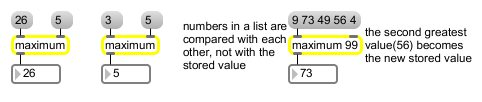# maximum

Output the greatest in a list of numbers

## Description

maximum watches an input stream for any number which is greater than its most recently set maximum, sets that new number as the maximum, and outputs it.

## Arguments

Name Type Opt Description
initial-comparison-value int or float opt Sets an initial value to be compared with numbers received in the left inlet. If the argument contains a decimal point, all numbers are compared as floats, and the output is a float. If there is no argument, the initial value is 0.

## Messages

 bang In left inlet: Sends the most recent output out the outlet again. int input [int] In left inlet: If the number is greater than the value currently stored in maximum, it is sent out the outlet. Otherwise, the stored value is sent out. (inlet1) comparison-value [int] In right inlet: The number is stored for comparison with subsequent numbers received in the left inlet. float input [float] Converted to int, unless there is a float argument, in which case all numbers are compared as floats. (inlet1) comparison-value [float] Converted to int, unless there is a float argument, in which case all numbers are compared as floats. list input [list] In left inlet: The numbers in the list are all compared to each other, and the greatest value is sent out the outlet. The value stored in maximum is replaced by the next greatest value in the list.The maximum object accepts lists of up to 256 elements.

## Output

float: The number received in the left inlet is compared with the value currently held by maximum (or numbers received as a list are compared with each other), and the greatest of the numbers is sent out the outlet. Floats are output only if there is an argument with a decimal point.
int: The number received in the left inlet is compared with the value currently held by maximum (or numbers received as a list are compared with each other), and the greatest of the numbers is sent out the outlet.

## ExamplesThe output is the greater of two numbers or the greatest in a list of numbers